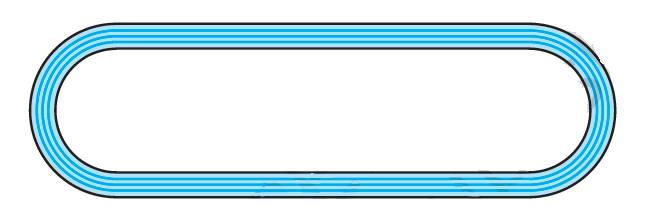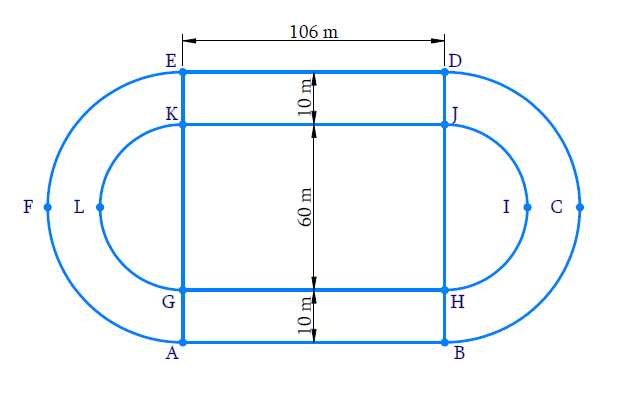In the verge of coronavirus pandemic, we are providing FREE access to our entire Online Curriculum to ensure Learning Doesn't STOP!

# Ex.12.3 Q8 Areas Related to Circles Solution - NCERT Maths Class 10

Go back to  'Ex.12.3'

## Question

Figure depicts a racing track whose left and right ends are semicircular. The distance between the two inner parallel line segments is $$\text{60 m}$$ and they are each $$\text{106 m}$$ long. If the track is $$\text{10 m}$$ wide, find:

(i) The distance around the track along its inner edge

(ii) The area of the track.Video Solution
Areas Related To Circles
Ex 12.3 | Question 8

## Text Solution

What is known?

(i) A racing track whose right and left ends are semicircular.

(ii) The distance between the $$2$$ inner parallel line segments is $$\text{60 m}$$ and they are $$\text{106 m}$$ long

(iii) Width of track $$\text{= 10 cm}$$

What is unknown?

(i) The distance around the track along its inner edge.

(ii) Area of the track.

Reasoning:(i) Draw a figure along with dimensions to visuals the track properly.

(ii) Visually the distance around the track along its inner edge.

$${ GH + {\rm{arc}}\, HIJ + JK + {\rm{arc}}\, KLG}$$

Where $$GH = JK = \text{106 m}$$

And arc $$HIJ = {\text{arc}}\, KLG =$$ circumference of semicircle with diameter $$\text{60 m.}$$

So, it can be easily found by substituting the required values.

(iii) To find area of track

Visually it’s clear that

Area of the tack = Area of rectangle $$ABHG \;+$$ Area of rectangle $$KJDE \;+$$ (Area of semicircle$$BCD\; –$$ Area of semicircle $$HIJ$$) + (Area of semicircle $$EFA\; –$$ Area of semicircle $$KLG$$)

Radii of semicircles $$HIJ$$ and $$KLG$$\begin{align} {\text{ = }}\frac{{60}}{{\text{2}}}{\text{ = 30 m}}\end{align}

Radii of semicircles $$BCD$$ and $$EFA =\text{ 30 m + 10 m = 40 m}$$

And $$JK = GH =\text{ 106 m}$$

And $$DJ = HB = \text{10 m}$$

Area of the track

=\left[ \begin{align} & GH\times HB+JK\times DJ+ \\ & \left( \frac{1}{2}\pi {{(40)}^{2}}-\frac{1}{2}{{(30)}^{2}} \right)+ \\ & \left( \frac{1}{2}\pi {{(40)}^{2}}-\frac{1}{2}{{(30)}^{2}} \right) \\ \end{align} \right]

Steps:Diameter of semicircle $$HIJ =$$ Diameter of semicircle $$KLG = \rm{60 m}$$

$$\therefore\;$$ their radius \begin{align}\left( {{r_1}} \right) = \frac{{60}}{2} = 30\,{\text{m}}\end{align}

(i) The distance around the track along its inner edge.

\begin{align}& =GH\!+\!\!arc HIJ \!+\!\!JK \!+\!\!arc KLG\\&= 106 + \frac{{2\pi {r_1}}}{2} + 106 + \frac{{2\pi {r_1}}}{2}\\ &= {106 + \pi \times 30 + 106 + \pi \times 30}\\&={ 212 + \frac{{1320}}{7}}\\ &= \frac{{1484 + 1320}}{7}\\ &= \frac{{2804}}{7}\,{\text{m}}\end{align}

(ii) Radius of semicircle $$BCD =$$ Radius of semicircle $$EFA$$

\begin{align}\left( {{r_2}} \right) &= 30\,\rm{m }+ 10m\\ &= 40\,\rm{m }\end{align}

Area of the tack $$=$$ Area of rectangle $$ABHG \,+$$ Area of rectangular $$KJDE \;+$$ (Area of semicircle $$BCD\;-$$ Area of semicircle $$HIJ$$) $$+$$(Area of semicircle $$EFA\; -$$ Area of semicircle $$KLG$$)

\begin{align} & =\left\{ \begin{array} & \left( 106\times 10 \right)+\left( 106\times 10 \right)+ \\ \left[ \frac{1}{2}\pi {{\left( 40 \right)}^{2}}-\frac{1}{2}\pi {{\left( 30 \right)}^{2}} \right]+ \\ \left[ \frac{1}{2}\pi {{\left( 40 \right)}^{2}}-\frac{1}{2}\pi {{\left( 30 \right)}^{2}} \right] \\\end{array} \right\} \\ & =\left\{ \begin{array} & 1060+1060+ \\ \left[ \frac{1}{2}\pi \left( 1600-900 \right) \right]+ \\ \left[ \frac{1}{2}\pi \left( 1600-900 \right) \right] \\ \end{array} \right\} \\ & =\left\{ \begin{array} & 1060+1060+ \\ \frac{\pi }{2}\times 700+\frac{\pi }{2}\times 700 \\ \end{array} \right\} \\ & =2120+700\pi \\ & =2120+700\times \frac{22}{7} \\ & =2120+2200 \\ & =4320{{\text{m}}^{\text{2}}} \\ \end{align}

Learn from the best math teachers and top your exams

• Live one on one classroom and doubt clearing
• Practice worksheets in and after class for conceptual clarity
• Personalized curriculum to keep up with school